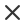過濾White gray abstract geometric background vector from polygons triangle vector illustration vector pattern triangular templatePREMIUMAbstract geometric background vector from polygons triangle background vector illustration vector pattern triangular template geometric samplePREMIUMWhite gray abstract geometric background vector from polygons triangle vector illustration vector pattern triangular templatePREMIUMAbstract geometric background vector from polygons triangle vector illustration vector pattern triangular templatePREMIUMSet karaoke microphone photo camera plastic bag of drug classic dart board and arrow cocktail alcohol drink and christmas lights icon vectorPREMIUMAbstract geometric background vector from polygons triangle background vector illustration vector pattern triangular template geometric samplePREMIUMWhite gray abstract geometric background vector from polygons triangle vector illustration vector pattern triangular templatePREMIUMWhite gray abstract geometric background vector from polygons triangle vector illustration vector pattern triangular templatePREMIUMWhite gray abstract geometric background vector from polygons triangle vector illustration vector pattern triangular templatePREMIUMQl monogram logo letter with triangle play button shape style on isolated background triangle monogram logo triangle play logo letterPREMIUMWhite gray abstract geometric background vector from polygons triangle vector illustration vector pattern triangular templatePREMIUMStructure molecule and communication dna atom neurons abstract polygonal structure with connecting dots and lines medical technology chemistry science background vector illustrationPREMIUMAbstract geometric background vector from polygons triangle background vector illustration vector pattern triangular template geometric samplePREMIUMBlack and white abstract circles circular spiral swirl twirl and whirl design elements stock vector illustration clip art graphicsPREMIUMWhite gray abstract geometric background vector from polygons triangle vector illustration vector pattern triangular templatePREMIUMWhite gray abstract geometric background vector from polygons triangle vector illustration vector pattern triangular templatePREMIUMAbstract geometric background vector from polygons triangle vector illustration vector pattern triangular templatePREMIUMAbstract geometric background vector from polygons triangle vector illustration vector pattern triangular templatePREMIUMAbstract geometric background vector from polygons triangle background vector illustration vector pattern triangular template geometric samplePREMIUMAbstract geometric background vector from polygons triangle background vector illustration vector pattern triangular template geometric samplePREMIUMWhite gray abstract geometric background vector from polygons triangle vector illustration vector pattern triangular templatePREMIUMWhite gray abstract geometric background vector from polygons triangle vector illustration vector pattern triangular templatePREMIUMAbstract geometric background vector from polygons triangle vector illustration vector pattern triangular templatePREMIUMAbstract geometric background vector from polygons triangle background vector illustration vector pattern triangular template geometric samplePREMIUMVector illustration of an abstract geometric background consisting of intersecting shapes with a shadow infographics 3d renderPREMIUMWhite backpack design front view college or school rucksack mockup vector illustration realistic youth pack of fabric for study or sport with shadowPREMIUMLoading related icon on background for graphic and web design simple illustration internet concept symbol for website button or mobile appPREMIUMWhite gray abstract geometric background vector from polygons triangle vector illustration vector pattern triangular templatePREMIUMWhite gray abstract geometric background vector from polygons triangle vector illustration vector pattern triangular templatePREMIUMAbstract geometric background vector from polygons triangle background vector illustration vector pattern triangular template geometric samplePREMIUMBottle drink water icon vector image can also be used for valentine love observances and holidays suitable for mobile apps web apps and print mediaPREMIUMAbstract geometric background vector from polygons triangle background vector illustration vector pattern triangular template geometric samplePREMIUMWhite gray abstract geometric background vector from polygons triangle vector illustration vector pattern triangular templatePREMIUMWhite gray abstract geometric background vector from polygons triangle vector illustration vector pattern triangular templatePREMIUMD letter logo in glass morphism style vector blurry translucent icon on white background frosted transparent blue and white emblem perfect for app modern design vibrant advertisingPREMIUMAbstract geometric background vector from polygons triangle background vector illustration vector pattern triangular templatePREMIUM